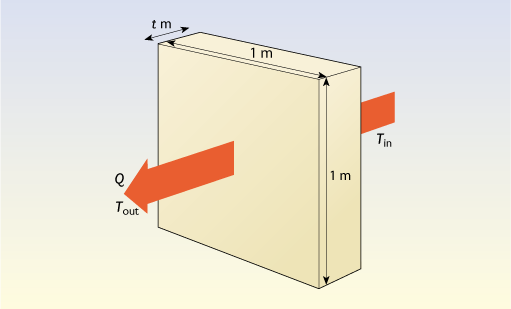Nature & Environment

### Become an OU studentEnergy in buildings

Start this free course now. Just create an account and sign in. Enrol and complete the course for a free statement of participation or digital badge if available.

# 2.2.4 Conductivity and U-values – the basics

U-values are used, as with windows, to describe the overall thermal performance of a building element such as a wall or roof. However these are likely to be made up of multiple layers of different materials (plaster, brick, insulation, etc.) each of which will have different thermal properties.

Thermal conductivities are useful for comparing the thermal properties of different materials (Tables 3 and 4), but for practical purposes we need to know the precise thermal resistance of a particular thickness of insulation material. This thermal resistance is analogous to electrical resistance using heat flow as current and temperature difference as voltage. The higher the resistance the greater the reduction in heat flow for a given temperature difference.

Consider a slab of a particular material t metres thick, with temperatures Tin and Tout on the two sides and a heat flow Q watts through each square metre (see Figure 9).Figure 9 Heat flow through a thin slab of material

The temperature difference per metre of thickness for this slab is (TinTout) / t, so it follows from the definition of thermal conductivity (λ) that the heat flow per square metre is:

• Q = (TinTout) × λ / t watts

The thermal resistance (R) of the slab is defined as the temperature difference divided by the heat flow per square metre:

• R = (TinTout) / Q m2 K W–1
• = t / λ m2 K W–1

The thicker the insulation, the greater the value of R.

Thus for a layer of insulation 100 mm (0.100 m) thick, with a thermal conductivity of 0.040 W m–1 K–1 the thermal resistance of the 100 mm layer will be:

• R = t / λ = 0.1 / 0.04 = 2.5 m2 K W-1

Finally, since the U-value of a slab is the heat flow divided by the temperature difference, R = 1 / U (and U = 1 / R).

Thus the U-value of this slab of insulation on its own is 1 / 2.5 = 0.4 W m-2 K−1.

An alternative way of looking at this problem is to say that a 1 metre-thick slab of this insulation will have a U-value on its own of 0.04 W m-2 K−1. Reducing the thickness by a factor of 10 to 100 mm will increase the heat flow and give a U-value of 0.4 W m-2 K−1.

Remember:

• U-values and thermal resistances are usually properties of whole building elements, while λ-values are properties of particular materials
• A good insulation material will have a low λ value
• A well insulated piece of building fabric will have a low U-value and a high thermal resistance.

## Activity 4

Just considering the materials alone, which has a higher thermal resistance: a piece of solid brick wall 250 mm thick with a conductivity of 0.77 W m−1 K−1 or 25 mm (one inch) of insulation with a conductivity of 0.040 W m−1 K−1?